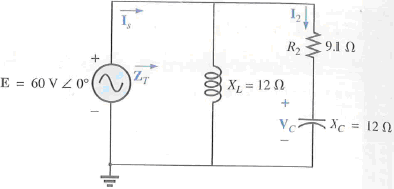Problem

# For the network in Fig. 1:a. Find the total impedance ZT.b. Find the current Is.c. Calcula...

For the network in Fig. 1:

a. Find the total impedance ZT.

b. Find the current Is.

c. Calculate I2 using the current divider rule.

d. Calculate VC using the voltage divider rule.

e. Calculate the average power delivered to the network.

FIG. 1#### Step-by-Step Solution

Request Professional Solution

#### Request Solution!

We need at least 9 more requests to produce the solution.

1 / 10 have requested this problem solution

The more requests, the faster the answer.

All students who have requested the solution will be notified once they are available.